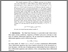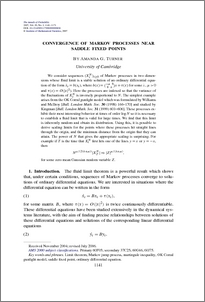# Convergence of Markov processes near saddle fixed points.

Turner, Amanda G. (2007) Convergence of Markov processes near saddle fixed points. Annals of Probability, 35 (3). pp. 1141-1171.Preview
PDF (http___projecteuclid.org_DPubS_Repository_1.0_Disseminate_handle=euclid.pdf)
http___projecteuclid.org_DPubS_Repository_1.0_Disseminate_handle=euclid.pdf

Download (283kB)

## Abstract

We consider sequences (XtN)t≥0 of Markov processes in two dimensions whose fluid limit is a stable solution of an ordinary differential equation of the form ẋt=b(xt), where for some λ, μ>0 and τ(x)=O(|x|2). Here the processes are indexed so that the variance of the fluctuations of XtN is inversely proportional to N. The simplest example arises from the OK Corral gunfight model which was formulated by Williams and McIlroy [Bull. London Math. Soc. 30 (1998) 166–170] and studied by Kingman [Bull. London Math. Soc. 31 (1999) 601–606]. These processes exhibit their most interesting behavior at times of order logN so it is necessary to establish a fluid limit that is valid for large times. We find that this limit is inherently random and obtain its distribution. Using this, it is possible to derive scaling limits for the points where these processes hit straight lines through the origin, and the minimum distance from the origin that they can attain. The power of N that gives the appropriate scaling is surprising. For example if T is the time that XtN first hits one of the lines y=x or y=−x, then Nμ/{(2(λ+μ))}|XTN| ⇒ |Z|μ/{(λ+μ)}, for some zero mean Gaussian random variable Z.

Item Type: Journal Article Annals of Probability RAE_import_type : Journal article RAE_uoa_type : Pure Mathematics /dk/atira/pure/researchoutput/libraryofcongress/qa Faculty of Science and Technology > Mathematics and Statistics 2395 ep_importer 01 Apr 2008 08:24 Yes Published 24 Aug 2019 00:32 https://eprints.lancs.ac.uk/id/eprint/2395

### Actions (login required)View Item